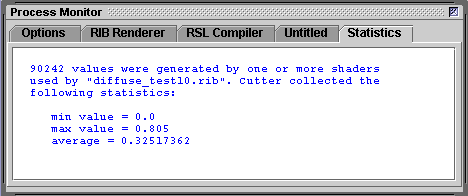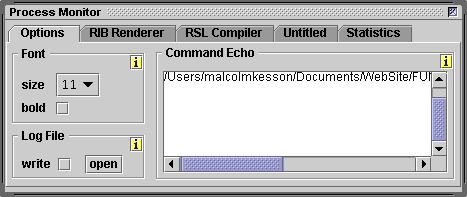#### Introduction

This tutorial provides code that demonstrates how values calculated in a displacement shader can be accessed by a surface shader - both shaders must be assigned to the same surface. For example, figure 1 shows the effect of a surface shader that querries the displacement values in order to assign white to the peaks of the bumps.Figure 1

The Pixar documentment that deals with this topic is,

#### Data Out/Data in

The code for a pair of shaders is shown below (listings 1 & 2). The first shader outputs a value called hump so that the surface shader can create the illusion of snow - figure 1. Although the shaders are far from sophisticated, they are a good starting point for developing cooperating shaders that could generate very interesting visual effects.

Listing 1

 ```displacement messageout(float Km = 0.1, freq = 4; output varying float hump = 0) { vector n = normalize(N); hump = noise(P * freq); /* Move the surface back to its original position */ hump = hump - 0.5; /* printf("%f\n", hump); */ P = P - n * hump * Km; N = calculatenormal(P); }```

Listing 2

 ```surface messagein(float Kd = 0.5, snow_ht = 0.0; color snow_color = 1, ground_color = color(0.466,0.388,0.309) ) { vector n = normalize(N), nf = faceforward(n, I); float height = 0; Oi = Os; /* Add snow according to the bump height */ if(displacement("hump", height) == 1) { float blend = smoothstep(snow_ht-0.05,snow_ht+0.05,height); surfcolor = mix(ground_color,snow_color,blend); } color diffusecolor = Kd * diffuse(nf); Ci = Oi * Cs * diffusecolor; }```

#### Output Values Min/Max

Because the output value in listing 1 is equal to `noise`() less 0.5 we know it will range in value from -0.5 to +0.5. However, output values are often based on a series of calculations and as such it can be difficult of anticipate their min and max values.

The `printf`() function can be used to print single or multiple values to the "terminal" from which the rib file that uses a shader is rendered. Cutter can capture the values in two ways.Figure 2

If a single value is written by the `printf`() statement, Cutter will generate some simple statistics, figure 2, that can be helpful in determining how a particular output value should be "manipulated" prior to being used by a surface shader.

Alternatively, if you wish to view a sequence of multiple values you can either render a rib file from the command prompt or you can direct Cutter (figure 3) to output a log file named rman_monitor.log. The log file will be saved in the same directory as cutter.jar.Figure 3

Once the range of values is known remove or comment the printf statement in the shader source code.